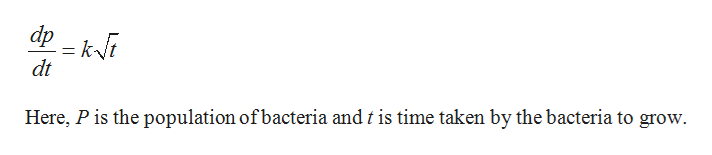# The rate of growth dP/dt of a population of bacteria is proportional to the square root of t where P is the population size and t is the time in days (0 ≤ t ≤ 10). That is, dP/dt = k√t The initial size of the population is 300. After 1 day the population has grown to 800. Estimate the population after 8 days.

Question
82 views

The rate of growth dP/dt of a population of bacteria is proportional to the square root of t where P is the population size and t is the time in days (0 ≤ t ≤ 10). That is, dP/dt = k√t The initial size of the population is 300. After 1 day the population has grown to 800. Estimate the population after 8 days.

check_circle

Step 1

The rate of growth dP/dt of a population of bacteria is proportional to the square root of t, where P is the population size and t is the time in days.

Step 2

Consider the rate at which the population of bacteria grow.help_outlineImage Transcriptionclosedp ki dt Here, P is the population of bacteria and t is time taken by the bacteria to grow fullscreen
Step 3

Integrate the growth to find the population...

### Want to see the full answer?

See Solution

#### Want to see this answer and more?

Solutions are written by subject experts who are available 24/7. Questions are typically answered within 1 hour.*

See Solution
*Response times may vary by subject and question.
Tagged in

### Calculus